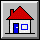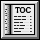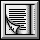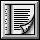`indexing`
```description: "Sequences of values, all of the same type or of a %
%conforming one, accessible through integer indices in a %
%contiguous interval"```
`class interface`
`ARRAY [G]`
`creation `
```make (minindex, maxindex: INTEGER)
-- Allocate array; set index interval to
-- minindex .. maxindex; set all values to default.
-- (Make array empty if minindex > maxindex.)
ensure
no_count: (minindex > maxindex) implies (count = 0);
count_constraint: (minindex <= maxindex) implies
(count = maxindex - minindex + 1)```
```make_from_array (a: ARRAY [G])
-- Initialize from the items of a.
-- (Useful in proper descendants of class ARRAY,
-- to initialize an array-like object from a manifest array.)```
`feature -- Initialization`
```make (minindex, maxindex: INTEGER)
-- Set index interval to minindex .. maxindex;
-- reallocate if necessary; set all values to default.
-- (Make array empty if minindex > maxindex.)
ensure
no_count: (minindex > maxindex) implies (count = 0);
count_constraint: (minindex <= maxindex) implies
(count = maxindex - minindex + 1)```
```make_from_array (a: ARRAY [G])
-- Initialize from the items of a; reallocate if
-- necessary. (Useful in proper descendants of
-- class ARRAY, to initialize an array-like object
-- from a manifest array.)```
`feature -- Access`
```entry (i: INTEGER): G
-- Entry at index i, if in index interval
-- (Redefinable synonym for item and infix "@".)
require
good_key: valid_index (i)```
```frozen item (i: INTEGER): G
-- Entry at index i, if in index interval
require
good_key: valid_index (i)```
```frozen infix "@" (i: INTEGER): G
-- Entry at index i, if in index interval
require
good_key: valid_index (i)```
`feature -- Measurement`
```count: INTEGER
-- Number of available indices```
```lower: INTEGER
-- Minimum index```
```upper: INTEGER
-- Maximum index```
`feature -- Comparison`
```is_equal (other: like Current): BOOLEAN
-- Is array made of the same items as other?
-- (Redefined from GENERAL.)```
`feature -- Status report`
```valid_index (i: INTEGER): BOOLEAN
-- Is i within the bounds of the array?```
`feature -- Element change`
```enter (v: G; i: INTEGER)
-- Repace i-th entry, if in index interval, by v.
-- (Redefinable synonym for put.)
require
good_key: valid_index (i)
ensure
inserted: item (i) = v```
```force (v: like item; i: INTEGER)
-- Assign item v to i-th entry.
-- Always applicable: resize the array if i falls out of
-- currently defined bounds; preserve existing items.
ensure
inserted: item (i) = v;
higher_count: count >= old count```
```frozen put (v: like item; i: INTEGER)
-- Replace i-th entry, if in index interval, by v.
require
good_key: valid_index (i)
ensure
inserted: item (i) = v```
`feature -- Resizing`
```resize (minindex, maxindex: INTEGER)
-- Rearrange array so that it can accommodate
-- indices down to minindex and up to maxindex.
-- Do not lose any previously entered item.
require
good_indices: minindex <= maxindex
ensure
no_low_lost: lower = minindex.min (old lower);
no_high_lost: upper = maxindex.max (old upper)```
`feature -- Conversion`
```to_c: POINTER
-- Address of actual sequence of values,
-- for passing to external (non-Eiffel) routines.```
`feature -- Duplication`
```copy (other: like Current)
-- Reinitialize by copying all the items of other.
-- (This is also used by clone.)
-- (From GENERAL.)```
`invariant`
```consistent_size: count = upper - lower + 1;
non_negative_count: count >= 0 ```
`end`

 Copyright © 1995, Nonprofit International Consortium for Eiffel mailto:nice@atlanta.twr.com Last Updated: 26 October 1997